We will discuss the following aspects. Please scroll down and start reading.

• Why need units ?
• International System of Units
• Definition of each Base Unit
• Derived quantities
• Units that are not part of the SI system

### Why need units?

Clear communication is very necessary for the safe and effective practice of anesthesia. You need to communicate matters precisely instead of being vague. Look at the patient note below:It doesn’t convey the actual amount of propofol given.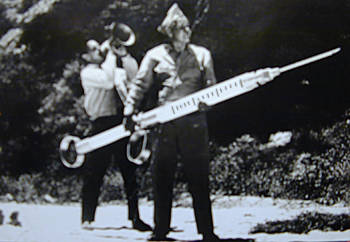On the other hand, if the same was written as :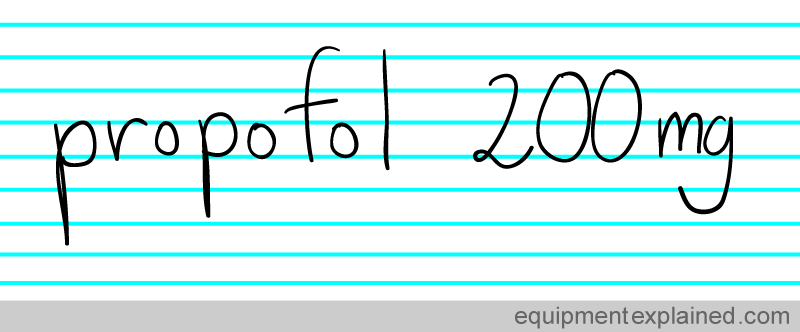There is no confusion. Wherever in the world you are , there is no doubt that the above note means that 200 mg of propofol was given. There is no confusion because everybody knows what a “mg” stands for. Standardized units make it easy for everyone to communicate accurately.

### International System of Units ( SI units )

The standardized system of measurement that is most well known and used in the world is the ” International System of Units”. It is NOT abbreviated as “ISOU”. Instead , the ” International System of Units” is abbreviated as ” SI Units” . The letters SI were taken from the French words that mean International System of Units ( it seems that historically the French were very involved with standardization).The SI system has “seven base” quantities. These are considered as basic quantities from which other quantities can be mathematically derived. The seven base quantities are: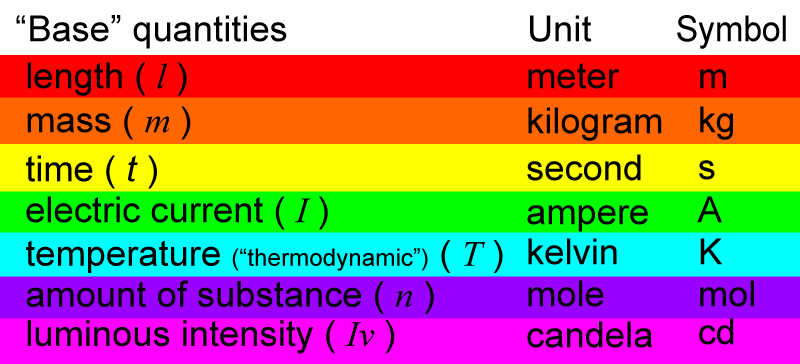The base quantities have abbreviated letters (in italics) to represent them:Each base quantity has a unit that is used to measure it.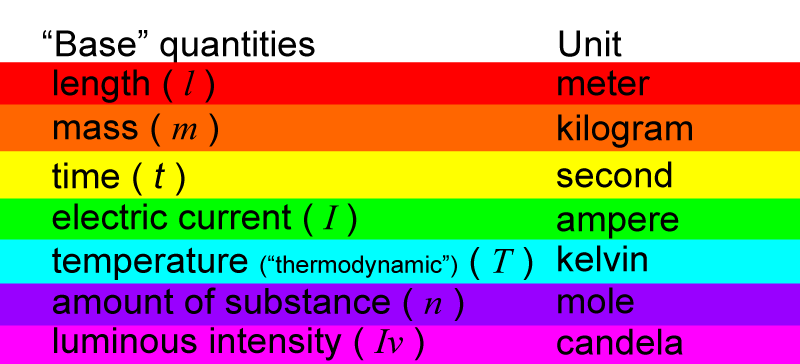And then, each unit of measurement has a symbol.E.g. Take the base quantity, mass.

This quantity, mass, can be represented by the letter ” m “. The unit of measurement used to measure mass is kilogram. Kilogram has a symbol to represent it, ” kg “.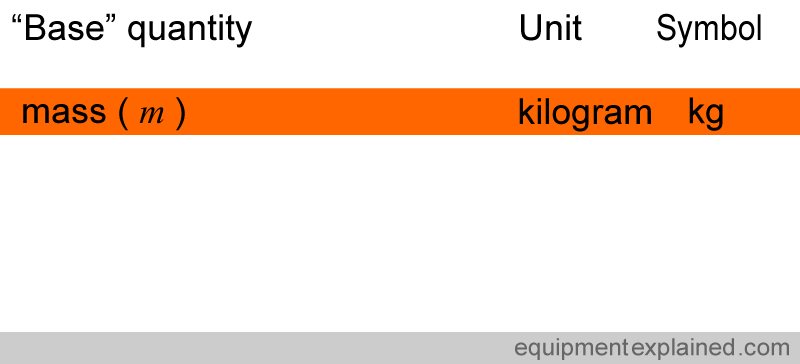When a symbol is derived from the name of a person, such as Kelvin and Ampere, the symbol is written with a capital letter.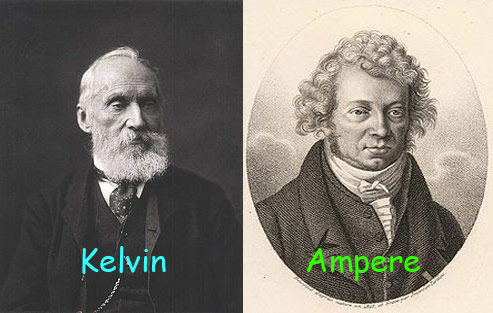So, in the list below, the symbols for ampere and kelvin are in capitol letters.### Definition of Units

Anaesthesia, is a science that demands accuracy. Many drugs that we administer are extremely potent and we need to be sure that the manufacture and administration of these drugs are done with quantities that we agree on precisely . The equipment we use for measurement must produce results that we all understand clearly .

The world of science has taken great efforts to make sure that the units of measurement are extremely precisely defined. They have tried not to rely on physical objects (e.g. “a meter stick” kept in some museum) to define something. The reason is that , such physical objects can be inadvertently damaged and cause great confusion. For example, imagine if there was a standard “meter stick” in the world and someone broke it into two halves in a fit of anger ! Would everyone become half as tall ?The only unit that still does rely on a physical object is the kilogram (discussed later).

One problem with making the definitions very accurate is that they are extremely complex. Please do not memorize them unless you have to. Just read these lightly , to get a rough idea.

Given below are the definitions of the seven base units of the SI system.

#### 1. DEFINITION OF METER ( unit of length )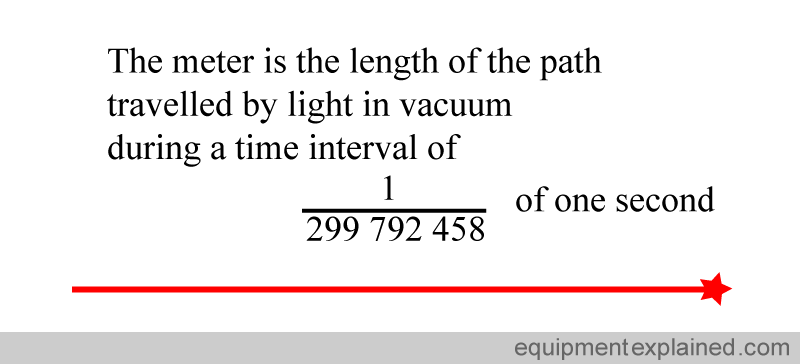#### 2. DEFINITION OF KILOGRAM ( unit of mass )

The definition of the kilogram is very unique. It is the only one where there is an actual object that represents it. Since the year 1889, the SI system defines the kilogram to be equal to the mass of what is called the “International Prototype Kilogram. This is a metallic cylinder, like the one below, kept very carefully in France.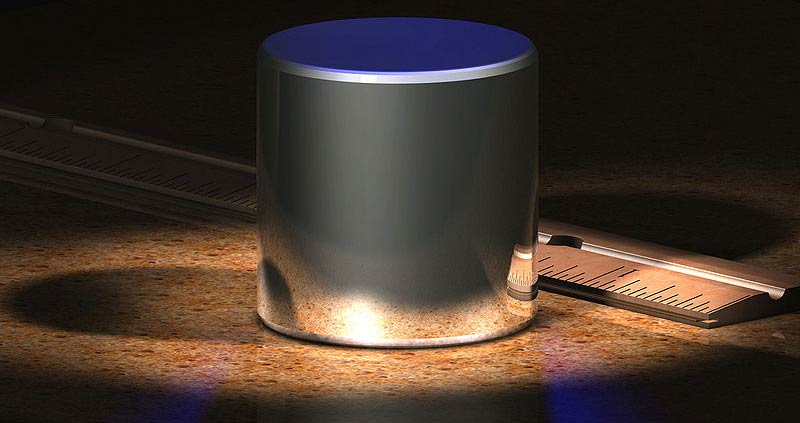It is kept very carefully in a vault, under multiple protective glass jars, as shown below.

They have to be careful with it, imagine what would happen to the world of science if someone dropped and broke it !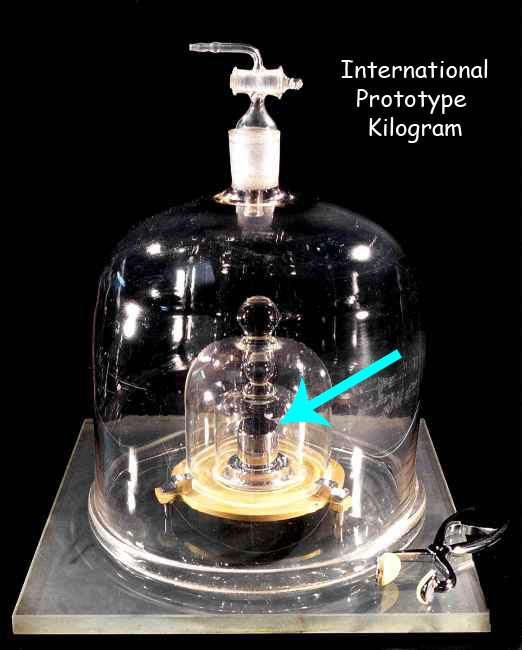#### 3. DEFINITION OF SECOND (unit of time)

The definition of the second is extremely complex. It involves the “oscillation” of two states of caesium atoms.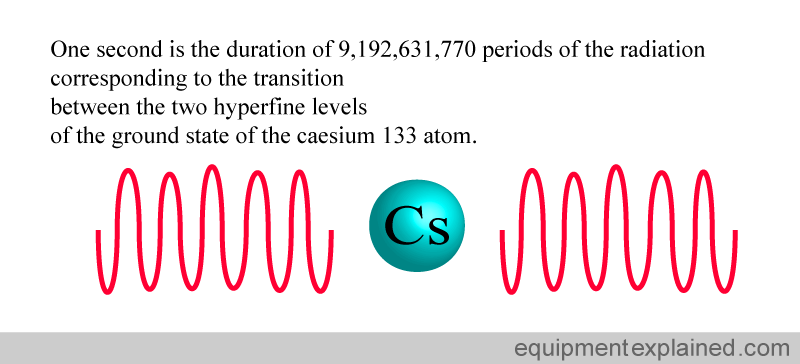Using atoms to define a second is an extremely accurate way of standardizing time. The clock below uses caesium atoms (atomic clock) to keep time. It is so accurate that it is expected to have an error of only one second for every 30 million years of operation.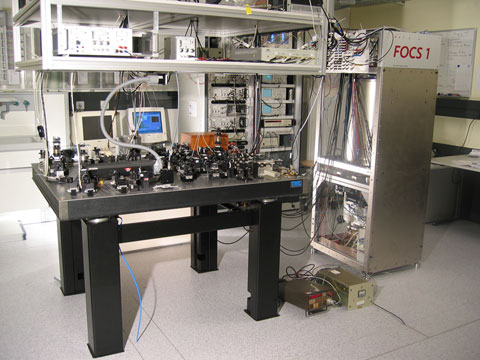#### 4. DEFINITION OF AMPERE (unit of current)

Wires that carry a current produce a magnetic field. Two wires carrying a current will attract each other because of the magnetic field that arises in each of them. This property is used to define a current of one ampere.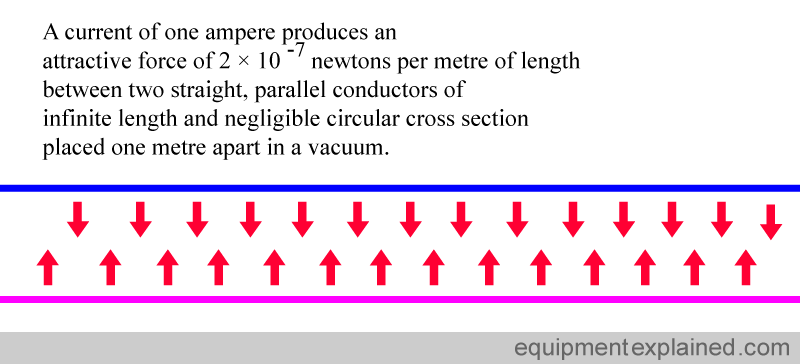#### 5. DEFINITION OF KELVIN (unit of temperature)

There is a very special temperature that is reproducible anywhere in the world. It is called the “triple point of water”. Water normally exists in one of three states: ice, liquid water, or water vapour. However, at a particular temperature, water in a vessel can exist in all three states at the same time (the three states being in “equilibrium” with each other). This happens ONLY at one particular temperature, which happens to be 273.16 kelvin. If the temperature is anything else, you cannot have water in these three states at the same time in “equilibrium” with each other. Since the triple point of water ( 273.16 K ) is reproducible in labs around in the world, it is used to define temperature.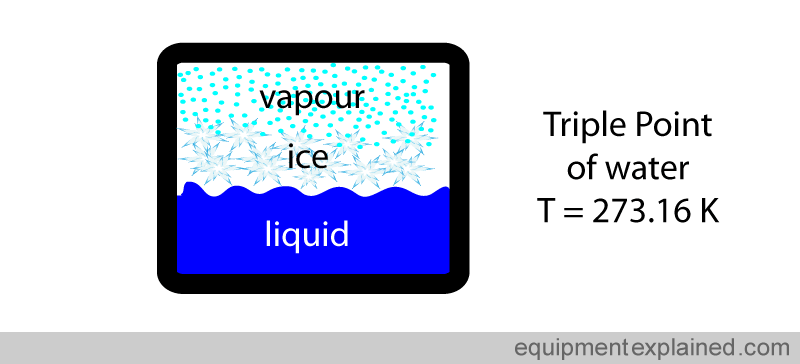The definition of the kelvin scale of temperature makes use of the unique temperature of the triple point of water.

First a temperature scale is made starting from zero kelvin (i.e. where there is no temperature) to the triple point of water , which is 273.16 kelvin.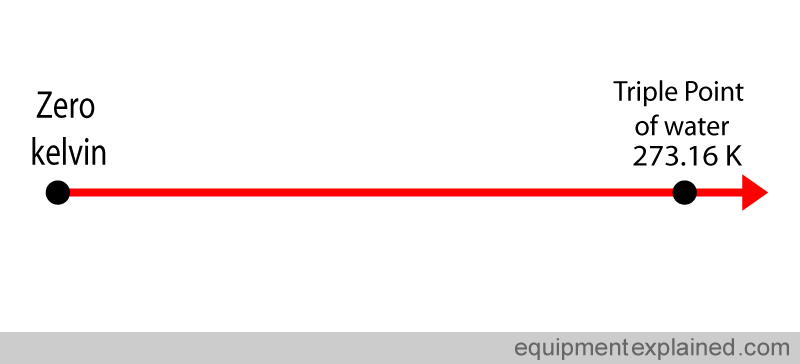This is divided into 273.16 equal parts.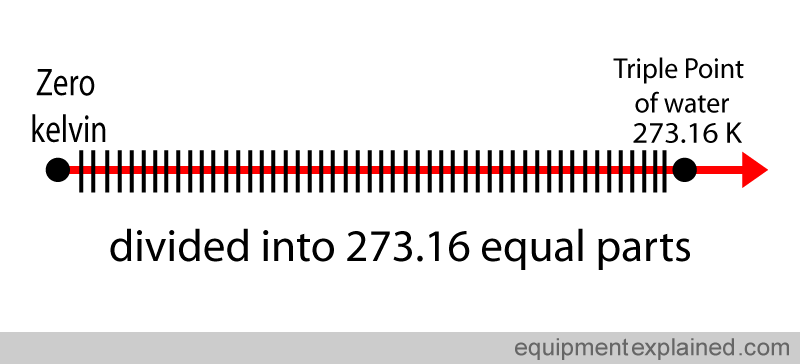Each of the divided parts equals one kelvin.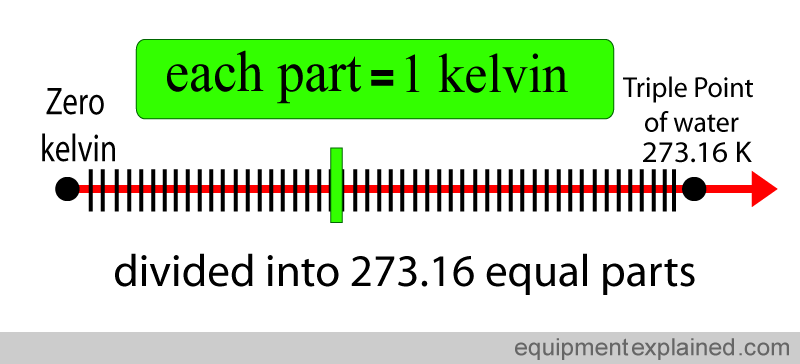The kelvin is not a common unit used in medicine. The most common temperature scale used in medicine is the Celsius scale. Zero degrees Celsius corresponds to 273 kelvin and – 273 Celsius corresponds to Zero Kelvin (see diagram below if you are confused !) ( to simplify this discussion, decimal places are being ignored. For an example, the triple point of water is not actually 0 degrees Celsius, but is 0.01 degrees Celsius).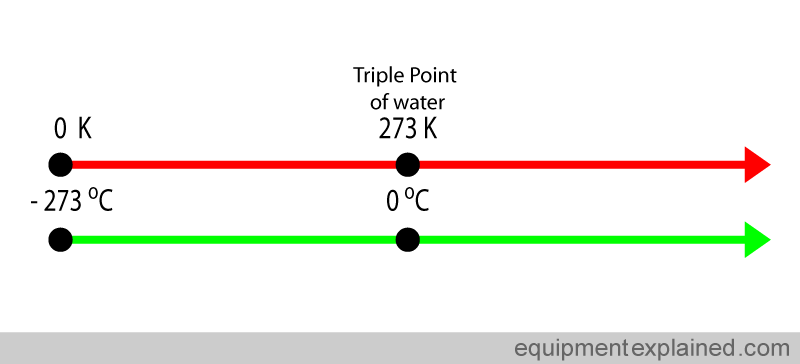Very conveniently, water boils at a nice 100 degrees Celsius.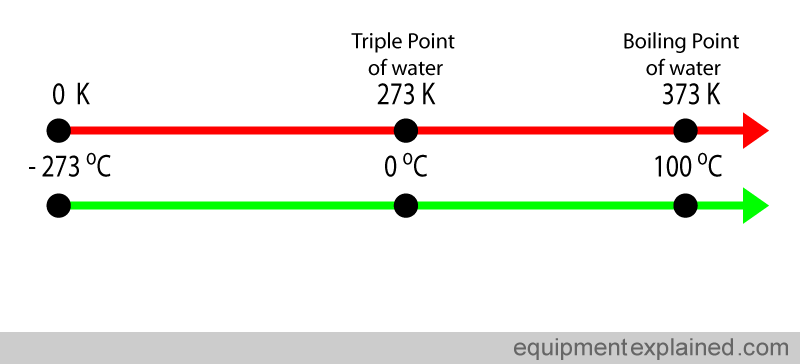Despite it not being a SI base unit, the Celsius scale is widely used in medicine and by the general public. Fortunately this does not create huge problems because the “size” of the Celsius degree and a kelvin degree are the same. Look at the diagram below. The difference between the triple point of water and the boiling of water is 100 degrees Celsius (i.e. 100 C – 0 C). If you look at the same points in the kelvin scale, the difference is also 100 degrees Kelvin (373 K – 273 K). So one degree in the kelvin scale and the Celsius scale are equal.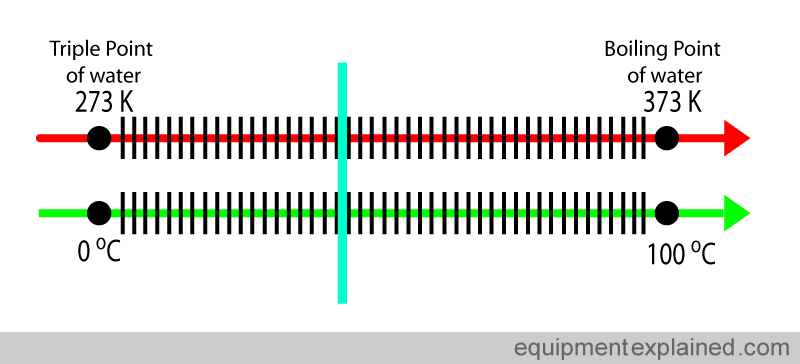Another scale that you may come across is the Fahrenheit scale. This is a scale mostly in use by the general public in the USA and a few other countries. The Fahrenheit scale is very different to the kelvin and Celsius scale and is not commonly used in scientific literature.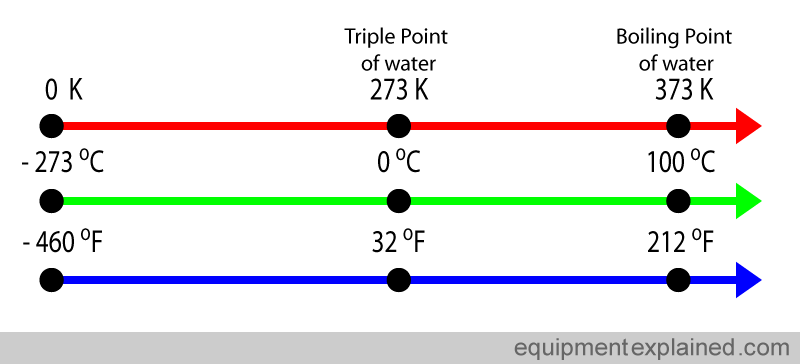#### 6. DEFINITION OF MOLE ( unit for amount of a substance )

We are not referring the the animal called mole …When you go shopping, you might choose to buy a “dozen” eggs. In the world of food, the term “dozen” is a convenient way of asking for twelve things.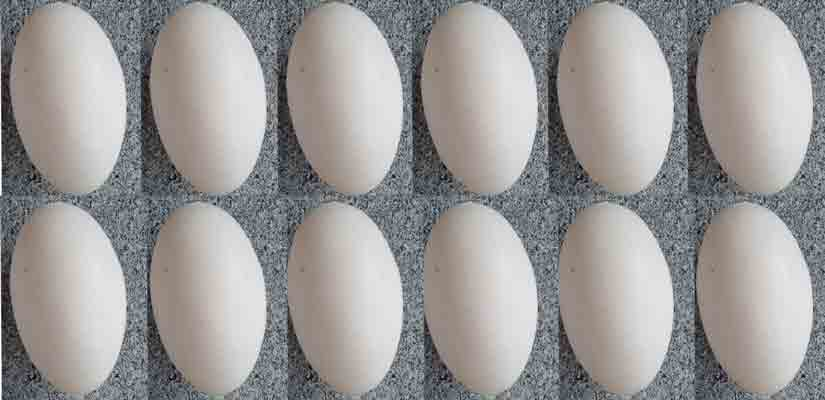In the world of science, they have chosen a “convenient” way of standardizing the quantity things by defining a quantity called a mole. Scientists use a unit much bigger than a “dozen”. In fact , they use a very much bigger number. The number they have chosen is:

602,300,000,000,000,000,000,000

This weird number was chosen to represent a mole because it is easy to standardize. It is the number of atomic particles in exactly 12 g of a type of carbon called “carbon 12”.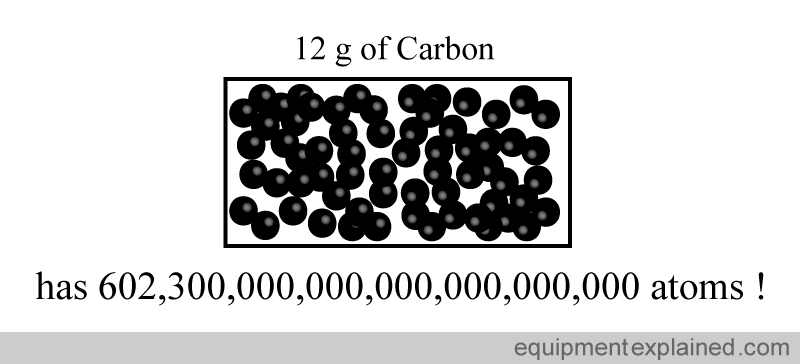A less dramatic way of expressing the same is to write it using mathematical notations: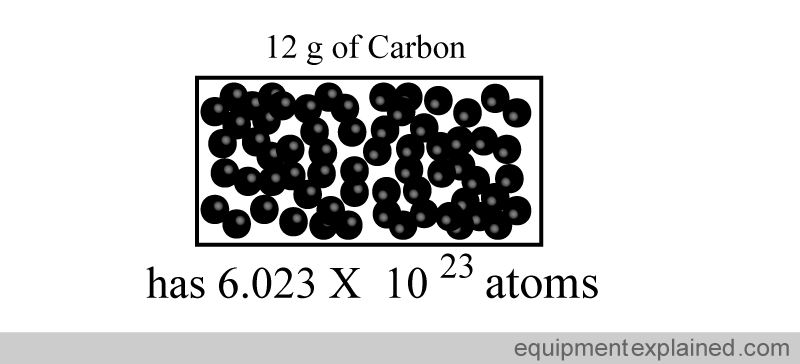“One mole” of a substance is defined as the amount of that substance that contains the same number of “particles” (e.g. atoms) as there are atoms in exactly “12 grams of carbon 12”.

So if you took “one mole” of sodium, it would have the same number of atoms as in 12 g of carbon: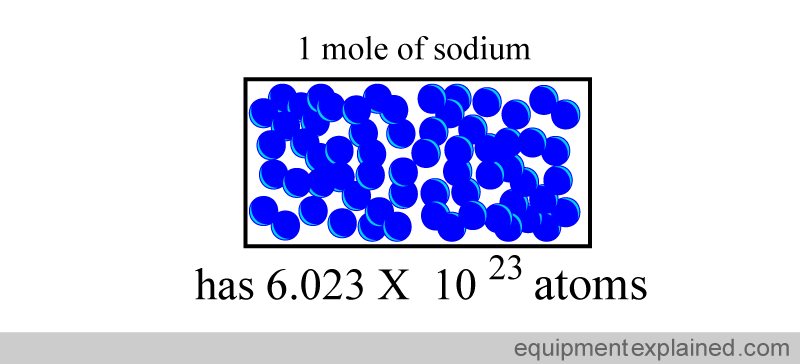Similarly, if you took “one mole” of potassium, it would have the same number of atoms as in 12 g of carbon: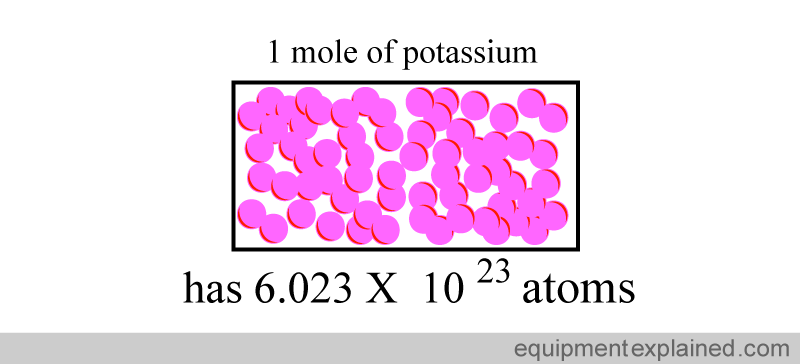In fact, if you took “one mole” of any substance, it would have the same number of atoms as in 12 g of carbon: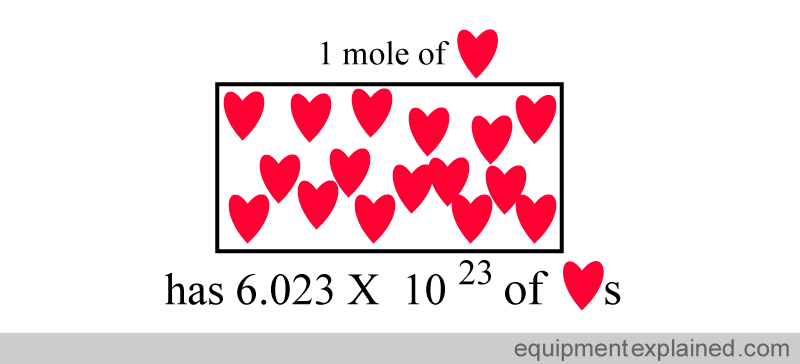Two moles of a substance has twice the number of particles as one mole.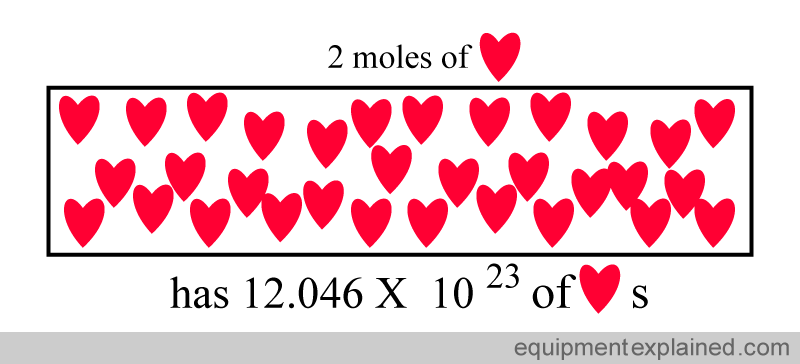So, remember that a mole is simply a standardized quantity.

#### 7. DEFINITION OF CANDELA (unit light intensity)

I will not even begin to explain this definition ( because I can’t):Fortunately, for practical purposes, one candela is the light emitted by one average candle.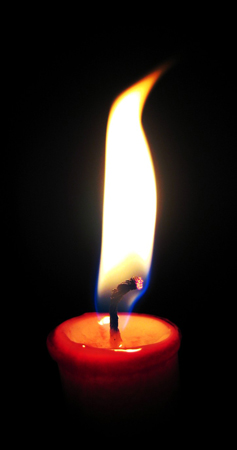### Derived Quantities & Special Names

There are quantities that are derived from the seven base units using equations. Here are some examples. Please note that there are much more derived quantities than this.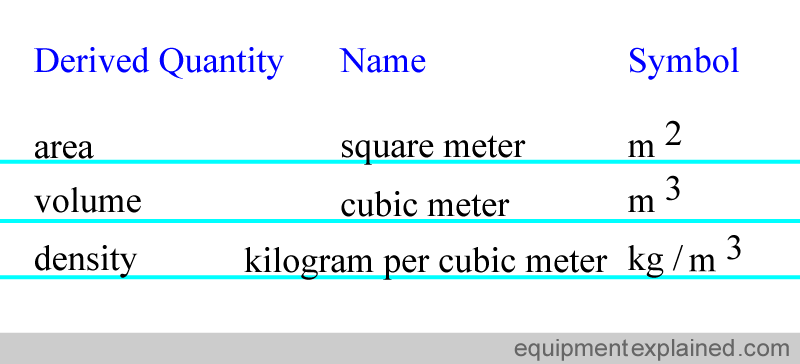For convenience, some of the derived quantities have been given special names. Here are some examples, but again, there are many more examples than shown.### Units that are not part of the SI systemThere are some measurement units that are not part of the SI system of units and “serious” scientists discourage their use. However, some of these are heavily used in medicine because they are convenient and are therefore “tolerated”. Examples include mmHg and Liter.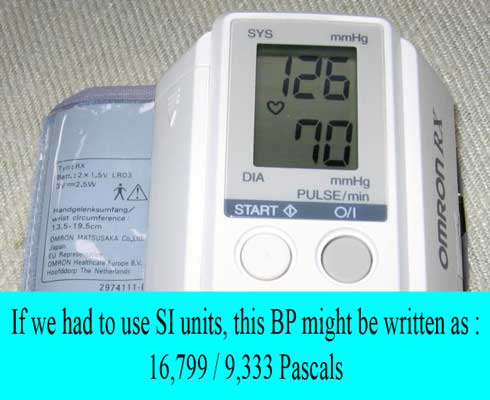We have now reached the end of our discussion on basic units. I hope it has given you a good introduction to the subject and will help you when you read further on this topic.  Hope to see you soon in another section. Bye for now.